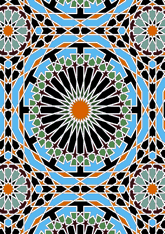# Tomb of Moulay Ishmael, Meknesdata194/MOR2443X

## Geometry

• The symmetry group of the tiling is *442 (p4m).
• All the internal angles of the constituent polygons are a multiple of 5°.
• Contains three regular two-pointed star polygons with vertex angle of 30°.
• Contains one regular hexagon.
• Contains one regular 12-pointed star polygon with vertex angle of 60°.
• Contains one regular 24-pointed star polygon with vertex angle of 30°.
• There are 19 non-regular reflective tiles (including 4 kites) and one reflective pair.
• The tiling satisfies the interlace condition and has one finite interlace and one infinite interlace with straight cross-overs.
• The tiling is edge-to-edge.
• As drawn, contains about 1009 polygons.

## References

Publications referenced: